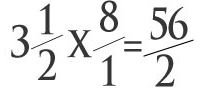# Multiplying Mixed Fractions the Easy Way

Page content

## Multiplying Mixed Numbers

Most people prefer to use mixed fractions rather than improper fractions, finding it easier to deal with something like 17 1/3 rather than 52/3. However, multiplying fractions while they’re in mixed form can be difficult; as it turns out, it’s much easier to simply convert them into improper fractions, multiply (or divide, for that matter), and then change the answer back to mixed form. Let’s take a look at how to do that.

## Changing Form: Mixed Fractions to Improper Fractions

Before we start, let’s review a few definitions. A mixed fraction is one that has both a whole and a fractional part, such as 3 1/2. An improper fraction is one where the numerator (the top part) is larger than the denominator (the bottom part), such as 7/2. Both of these examples represent the same number (3.5); it’s just written differently.

Changing fractions from mixed to improper form is pretty easy; you don’t even need to find a least common denominator, like you would if you were comparing or subtracting fractions.

Let’s look at what a whole number represents. Suppose that 3.5 above represents how many pizzas we have left over from a party; we have 3 whole pizzas, plus half of a pizza. We can write that at 3 1/2 (three and one half) or 7/2 (seven halves, which form three wholes plus one more half).

Recall that anything divided by itself equals one: 2/2 = 1, 3/3 = 1, etc. To convert from mixed to improper form, start by changing the whole number part into a fraction. Continuing with our example, we multiply 3 by 2 to get 6/2; 6/2 is the same as three. We now have two fractions with like denominators, so we can add: 6/2 + 1/2 = 7/2. Another way of saying this is to multiply the whole number by the denominator, add the numerator, and place the result over the original denominator.

## Time for the Multiplication!So the first step in multiplying mixed fractions was to change both numbers into improper fractions. Finding the product of two fractions is easy: just multiply the numerators, then multiply the denominators. For example, if I was to multiply 2/3 by 5/7, I would get 2*5 over 3*7, or 10/21.

With improper fractions, the same rule applies, so multiplying 3/2 by 7/5 gives 21/10. To change this back into a mixed fraction, I remember that every 10/10 is one whole; I can take 10 out of 21 twice, with one left over, so 21/10 is the same as 2 1/10.

Going back to our pizzas, we found that we had 7/2 pizzas. Suppose each pizza has 8 slices, which we can write as 8/1; then there are (7*8)/(2*1) = 56/2 = 28 slices of pizza left!